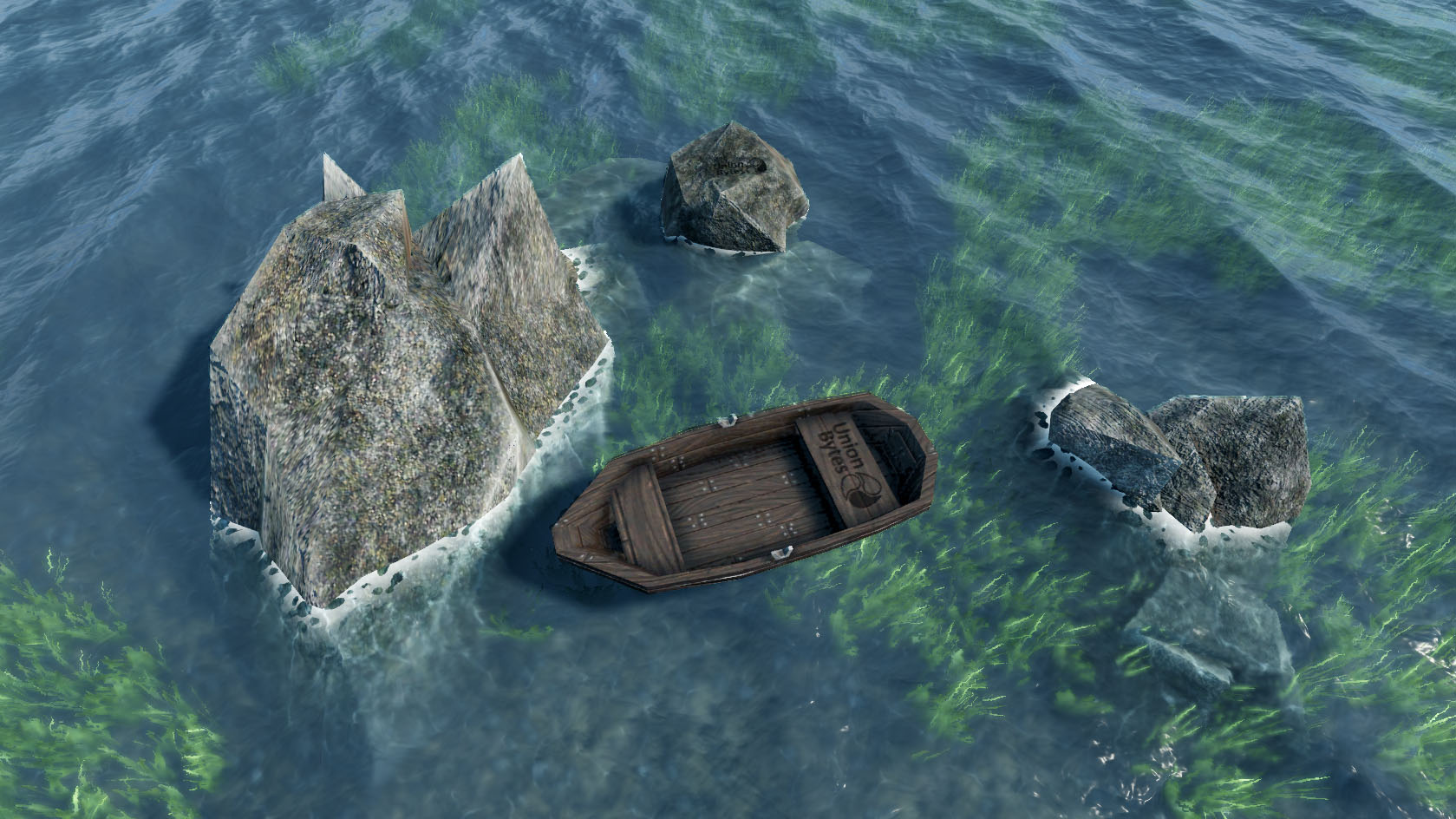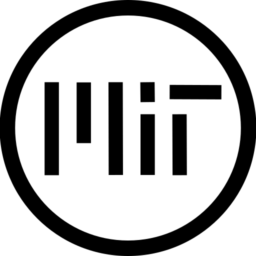# Realistic Water

Beautiful realistic water shader from UnionBytes.

Textures are available here.

``````/*
Realistic Water Shader for Godot 3.4
Modified to work with Godot 3.4 with thanks to jmarceno.
Copyright (c) 2019 UnionBytes, Achim Menzel (alias AiYori)
Permission is hereby granted, free of charge, to any person obtaining a copy of this software and associated documentation files (the "Software"), to deal in the Software without restriction, including without limitation the rights to use, copy, modify, merge, publish, distribute, sublicense, and/or sell copies of the Software, and to permit persons to whom the Software is furnished to do so, subject to the following conditions:
The above copyright notice and this permission notice shall be included in all copies or substantial portions of the Software.
THE SOFTWARE IS PROVIDED "AS IS", WITHOUT WARRANTY OF ANY KIND, EXPRESS OR IMPLIED, INCLUDING BUT NOT LIMITED TO THE WARRANTIES OF MERCHANTABILITY, FITNESS FOR A PARTICULAR PURPOSE AND NONINFRINGEMENT. IN NO EVENT SHALL THE AUTHORS OR COPYRIGHT HOLDERS BE LIABLE FOR ANY CLAIM, DAMAGES OR OTHER LIABILITY, WHETHER IN AN ACTION OF CONTRACT, TORT OR OTHERWISE, ARISING FROM, OUT OF OR IN CONNECTION WITH THE SOFTWARE OR THE USE OR OTHER DEALINGS IN THE SOFTWARE.
-- UnionBytes
*/

// For this shader min. GODOT 3.1.1 is required, because 3.1 has a depth buffer bug!
render_mode 	cull_back,diffuse_burley,specular_schlick_ggx, blend_mix;

// Wave settings:
uniform float	wave_speed		 = 0.5; // Speed scale for the waves
uniform vec4	wave_a			 = vec4(1.0, 1.0, 0.35, 3.0); 	// xy = Direction, z = Steepness, w = Length
uniform	vec4	wave_b			 = vec4(1.0, 0.6, 0.30, 1.55);	// xy = Direction, z = Steepness, w = Length
uniform	vec4	wave_c			 = vec4(1.0, 1.3, 0.25, 0.9); 	// xy = Direction, z = Steepness, w = Length

// Surface settings:
uniform vec2 	sampler_scale 	 = vec2(0.25, 0.25); 			// Scale for the sampler
uniform vec2	sampler_direction= vec2(0.05, 0.04); 			// Direction and speed for the sampler offset

uniform sampler2D uv_sampler : hint_aniso; 						// UV motion sampler for shifting the normalmap
uniform vec2 	uv_sampler_scale = vec2(0.25, 0.25); 			// UV sampler scale
uniform float 	uv_sampler_strength = 0.04; 					// UV shifting strength

uniform sampler2D normalmap_a_sampler : hint_normal;			// Normalmap sampler A
uniform sampler2D normalmap_b_sampler : hint_normal;			// Normalmap sampler B

uniform sampler2D foam_sampler : hint_black;					// Foam sampler
uniform float 	foam_level 		 = 0.5;							// Foam level -> distance from the object (0.0 - 0.5)

// Volume settings:
uniform float 	refraction 		 = 0.075;						// Refraction of the water

uniform vec4 	color_deep : hint_color;						// Color for deep places in the water, medium to dark blue
uniform vec4 	color_shallow : hint_color;						// Color for lower places in the water, bright blue - green
uniform float 	beers_law		 = 2.0;							// Beers law value, regulates the blending size to the deep water level
uniform float 	depth_offset	 = -0.75;						// Offset for the blending

// Projector for the water caustics:
uniform mat4	projector;										// Projector matrix, mostly the matric of the sun / directlight
uniform sampler2DArray caustic_sampler : hint_black;			// Caustic sampler, (Texture array with 16 Textures for the animation)

// Vertex -> Fragment:
varying float 	vertex_height;									// Height of the water surface
varying vec3 	vertex_normal;									// Vertex normal -> Needed for refraction calculation
varying vec3 	vertex_binormal;								// Vertex binormal -> Needed for refraction calculation
varying vec3 	vertex_tangent;									// Vertex tangent -> Needed for refraction calculation

varying mat4 	inv_mvp; 										// Inverse ModelViewProjection matrix -> Needed for caustic projection

// Wave function:
vec4 wave(vec4 parameter, vec2 position, float time, inout vec3 tangent, inout vec3 binormal)
{
float	wave_steepness	 = parameter.z;
float	wave_length		 = parameter.w;

float	k				 = 2.0 * 3.14159265359 / wave_length;
float 	c 				 = sqrt(9.8 / k);
vec2	d				 = normalize(parameter.xy);
float 	f 				 = k * (dot(d, position) - c * time);
float 	a				 = wave_steepness / k;

tangent			+= normalize(vec3(1.0-d.x * d.x * (wave_steepness * sin(f)), d.x * (wave_steepness * cos(f)), -d.x * d.y * (wave_steepness * sin(f))));
binormal		+= normalize(vec3(-d.x * d.y * (wave_steepness * sin(f)), d.y * (wave_steepness * cos(f)), 1.0-d.y * d.y * (wave_steepness * sin(f))));

return vec4(d.x * (a * cos(f)), a * sin(f) * 0.25, d.y * (a * cos(f)), 0.0);
}

void vertex()
{
float	time			 = TIME * wave_speed;

vec4	vertex			 = vec4(VERTEX, 1.0);
vec3	vertex_position  = (WORLD_MATRIX * vertex).xyz;

vec3 tang = vec3(0.0, 0.0, 0.0);
vec3 bin = vec3(0.0, 0.0, 0.0);

vertex 			+= wave(wave_a, vertex_position.xz, time, tang, bin);
vertex 			+= wave(wave_b, vertex_position.xz, time, tang, bin);
vertex 			+= wave(wave_c, vertex_position.xz, time, tang, bin);

vertex_tangent 	 = tang;
vertex_binormal  = bin;

vertex_position  = vertex.xyz;

vertex_height	 = (PROJECTION_MATRIX * MODELVIEW_MATRIX * vertex).z;

TANGENT			 = vertex_tangent;
BINORMAL		 = vertex_binormal;
vertex_normal	 = normalize(cross(vertex_binormal, vertex_tangent));
NORMAL			 = vertex_normal;

UV				 = vertex.xz * sampler_scale;

VERTEX			 = vertex.xyz;

inv_mvp = inverse(PROJECTION_MATRIX * MODELVIEW_MATRIX);
}

void fragment()
{
// Calculation of the UV with the UV motion sampler
vec2	uv_offset 					 = sampler_direction * TIME;
vec2 	uv_sampler_uv 				 = UV * uv_sampler_scale + uv_offset;
vec2	uv_sampler_uv_offset 		 = uv_sampler_strength * texture(uv_sampler, uv_sampler_uv).rg * 2.0 - 1.0;
vec2 	uv 							 = UV + uv_sampler_uv_offset;

// Normalmap:
vec3 	normalmap					 = texture(normalmap_a_sampler, uv - uv_offset*2.0).rgb * 0.75;		// 75 % sampler A
normalmap 					+= texture(normalmap_b_sampler, uv + uv_offset).rgb * 0.25;			// 25 % sampler B

// Refraction UV:
vec3	ref_normalmap				 = normalmap * 2.0 - 1.0;
ref_normalmap				 = normalize(vertex_tangent*ref_normalmap.x + vertex_binormal*ref_normalmap.y + vertex_normal*ref_normalmap.z);
vec2 	ref_uv						 = SCREEN_UV + (ref_normalmap.xy * refraction) / vertex_height;

// Ground depth:
float 	depth_raw					 = texture(DEPTH_TEXTURE, ref_uv).r * 2.0 - 1.0;
float	depth						 = PROJECTION_MATRIX / (depth_raw + PROJECTION_MATRIX);

float 	depth_blend 				 = exp((depth+VERTEX.z + depth_offset) * -beers_law);
depth_blend 				 = clamp(1.0-depth_blend, 0.0, 1.0);
float	depth_blend_pow				 = clamp(pow(depth_blend, 2.5), 0.0, 1.0);

// Ground color:
vec3 	screen_color 				 = textureLod(SCREEN_TEXTURE, ref_uv, depth_blend_pow * 2.5).rgb;

vec3 	dye_color 					 = mix(color_shallow.rgb, color_deep.rgb, depth_blend_pow);
vec3	color 						 = mix(screen_color*dye_color, dye_color*0.25, depth_blend_pow*0.5);

// Caustic screen projection
vec4 	caustic_screenPos 			 = vec4(ref_uv*2.0-1.0, depth_raw, 1.0);
vec4 	caustic_localPos 			 = inv_mvp * caustic_screenPos;
caustic_localPos			 = vec4(caustic_localPos.xyz/caustic_localPos.w, caustic_localPos.w);

vec2 	caustic_Uv 					 = caustic_localPos.xz / vec2(1024.0) + 0.5;
vec4	caustic_color				 = texture(caustic_sampler, vec3(caustic_Uv*300.0, mod(TIME*14.0, 16.0)));

color 						*= 1.0 + pow(caustic_color.r, 1.50) * (1.0-depth_blend) * 6.0;

// Foam:
if(depth + VERTEX.z < vertex_height-0.1)
{
float foam_noise = clamp(pow(texture(foam_sampler, (uv*4.0) - uv_offset).r, 10.0)*40.0, 0.0, 0.2);
float foam_mix = clamp(pow((1.0-(depth + VERTEX.z) + foam_noise), 8.0) * foam_noise * 0.4, 0.0, 1.0);
color = mix(color, vec3(1.0), foam_mix);
}

// Set all values:
ALBEDO = color;
METALLIC = 0.1;
ROUGHNESS = 0.2;
SPECULAR = 0.2 + depth_blend_pow * 0.4;
NORMALMAP = normalmap;
NORMALMAP_DEPTH = 1.25;
}``````
###### Tags
caustic, environment, foam, refraction, wavesThe shader code and all code snippets in this post are under MIT license and can be used freely. Images and videos, and assets depicted in those, do not fall under this license. For more info, see our License terms.

### Low poly water

Subscribe
Notify ofInline Feedbacksmrlonely
2 years ago

This is really, really great thanks 🙂mac
2 years ago

are you planning to update the shader to work with 3.4?jmarceno
2 years ago

Not the author, but I updated it to work with 3.4.Sslaxx
1 year ago

You may want to note that the GitHub repository for the water has been updated with jmarceno’s changes.Rasmus
1 year ago

I seem to have a problem with Refraction where it makes a line beneath all objects above water and refracts that line (also adds the foam). Any idea why?kmare
1 year ago